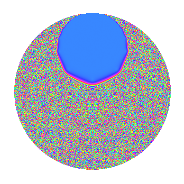# Properties

 Label 3.40.a.bLevel 3 Weight 40 Character orbit 3.a Self dual Yes Analytic conductor 28.902 Analytic rank 0 Dimension 3 CM No Inner twists 1

# Related objects

## Newspace parameters

 Level: $$N$$ = $$3$$ Weight: $$k$$ = $$40$$ Character orbit: $$[\chi]$$ = 3.a (trivial)

## Newform invariants

 Self dual: Yes Analytic conductor: $$28.9018654169$$ Analytic rank: $$0$$ Dimension: $$3$$ Coefficient field: $$\mathbb{Q}[x]/(x^{3} - \cdots)$$ Coefficient ring: $$\Z[a_1, a_2]$$ Coefficient ring index: $$2^{8}\cdot 3^{6}\cdot 5\cdot 7\cdot 13$$ Fricke sign: $$1$$ Sato-Tate group: $\mathrm{SU}(2)$

## $q$-expansion

Coefficients of the $$q$$-expansion are expressed in terms of a basis $$1,\beta_1,\beta_2$$ for the coefficient ring described below. We also show the integral $$q$$-expansion of the trace form.

 $$f(q)$$ $$=$$ $$q + ( 177858 - \beta_{1} ) q^{2} -1162261467 q^{3} + ( 319147551244 - 227081 \beta_{1} + \beta_{2} ) q^{4} + ( -17793991314810 + 12092638 \beta_{1} + 54 \beta_{2} ) q^{5} + ( -206717499997686 + 1162261467 \beta_{1} ) q^{6} + ( -525770892074176 - 12596523938 \beta_{1} + 56758 \beta_{2} ) q^{7} + ( 149112778455452232 - 182657330678 \beta_{1} + 533574 \beta_{2} ) q^{8} + 1350851717672992089 q^{9} +O(q^{10})$$ $$q +(177858 - \beta_{1}) q^{2} -1162261467 q^{3} +(319147551244 - 227081 \beta_{1} + \beta_{2}) q^{4} +(-17793991314810 + 12092638 \beta_{1} + 54 \beta_{2}) q^{5} +(-206717499997686 + 1162261467 \beta_{1}) q^{6} +(-525770892074176 - 12596523938 \beta_{1} + 56758 \beta_{2}) q^{7} +(149112778455452232 - 182657330678 \beta_{1} + 533574 \beta_{2}) q^{8} +1350851717672992089 q^{9} +(-13289593742193286260 - 3323523968902 \beta_{1} + 4457984 \beta_{2}) q^{10} +(-$$$$17\!\cdots\!80$$$$+ 2617390166972 \beta_{1} - 42093972 \beta_{2}) q^{11} +(-$$$$37\!\cdots\!48$$$$+ 263927496187827 \beta_{1} - 1162261467 \beta_{2}) q^{12} +($$$$18\!\cdots\!30$$$$- 3296990601565580 \beta_{1} + 2302171012 \beta_{2}) q^{13} +($$$$10\!\cdots\!84$$$$- 22915977673056320 \beta_{1} + 29992453632 \beta_{2}) q^{14} +($$$$20\!\cdots\!70$$$$- 14054807181779946 \beta_{1} - 62762119218 \beta_{2}) q^{15} +($$$$40\!\cdots\!12$$$$- 247808314916500164 \beta_{1} - 203561787228 \beta_{2}) q^{16} +($$$$24\!\cdots\!86$$$$- 1432579830459091908 \beta_{1} + 73008839916 \beta_{2}) q^{17} +($$$$24\!\cdots\!62$$$$- 1350851717672992089 \beta_{1}) q^{18} +(-$$$$35\!\cdots\!08$$$$- 3709293048070314324 \beta_{1} + 14019280007196 \beta_{2}) q^{19} +($$$$10\!\cdots\!20$$$$+ 4685500071252208714 \beta_{1} - 24996949090938 \beta_{2}) q^{20} +($$$$61\!\cdots\!92$$$$+ 14640454391280497046 \beta_{1} - 65967636343986 \beta_{2}) q^{21} +(-$$$$33\!\cdots\!08$$$$+$$$$19\!\cdots\!84$$$$\beta_{1} - 15518897927168 \beta_{2}) q^{22} +($$$$13\!\cdots\!88$$$$+ 71070379474158455740 \beta_{1} + 864912659360364 \beta_{2}) q^{23} +(-$$$$17\!\cdots\!44$$$$+$$$$21\!\cdots\!26$$$$\beta_{1} - 620152499993058 \beta_{2}) q^{24} +(-$$$$39\!\cdots\!25$$$$- 37236871224450578840 \beta_{1} - 3116005674041720 \beta_{2}) q^{25} +($$$$30\!\cdots\!92$$$$-$$$$29\!\cdots\!78$$$$\beta_{1} + 4002589901546496 \beta_{2}) q^{26} -$$$$15\!\cdots\!63$$$$q^{27} +($$$$21\!\cdots\!52$$$$-$$$$16\!\cdots\!88$$$$\beta_{1} + 905414279433792 \beta_{2}) q^{28} +($$$$24\!\cdots\!54$$$$-$$$$60\!\cdots\!54$$$$\beta_{1} + 10883586973384674 \beta_{2}) q^{29} +($$$$15\!\cdots\!20$$$$+$$$$38\!\cdots\!34$$$$\beta_{1} - 5181343023702528 \beta_{2}) q^{30} +(-$$$$12\!\cdots\!52$$$$+$$$$13\!\cdots\!26$$$$\beta_{1} - 51025473408711938 \beta_{2}) q^{31} +($$$$12\!\cdots\!04$$$$+$$$$16\!\cdots\!32$$$$\beta_{1} - 107917356575846952 \beta_{2}) q^{32} +($$$$20\!\cdots\!60$$$$-$$$$30\!\cdots\!24$$$$\beta_{1} + 48924201648576924 \beta_{2}) q^{33} +($$$$12\!\cdots\!48$$$$-$$$$34\!\cdots\!14$$$$\beta_{1} + 1454956528831466496 \beta_{2}) q^{34} +($$$$91\!\cdots\!40$$$$+$$$$42\!\cdots\!08$$$$\beta_{1} - 2423830555879147836 \beta_{2}) q^{35} +($$$$43\!\cdots\!16$$$$-$$$$30\!\cdots\!09$$$$\beta_{1} + 1350851717672992089 \beta_{2}) q^{36} +($$$$96\!\cdots\!74$$$$-$$$$33\!\cdots\!92$$$$\beta_{1} - 3950416425949998144 \beta_{2}) q^{37} +($$$$24\!\cdots\!64$$$$-$$$$22\!\cdots\!08$$$$\beta_{1} + 8006104235315837952 \beta_{2}) q^{38} +(-$$$$21\!\cdots\!10$$$$+$$$$38\!\cdots\!60$$$$\beta_{1} - 2675724657691994604 \beta_{2}) q^{39} +($$$$51\!\cdots\!00$$$$+$$$$19\!\cdots\!20$$$$\beta_{1} - 14797692611200750940 \beta_{2}) q^{40} +(-$$$$46\!\cdots\!42$$$$+$$$$18\!\cdots\!28$$$$\beta_{1} + 41049028078685916228 \beta_{2}) q^{41} +(-$$$$12\!\cdots\!28$$$$+$$$$26\!\cdots\!40$$$$\beta_{1} - 34859073157257798144 \beta_{2}) q^{42} +(-$$$$80\!\cdots\!76$$$$-$$$$20\!\cdots\!56$$$$\beta_{1} + 42956960985072135700 \beta_{2}) q^{43} +(-$$$$71\!\cdots\!04$$$$+$$$$48\!\cdots\!16$$$$\beta_{1} -$$$$17\!\cdots\!72$$$$\beta_{2}) q^{44} +(-$$$$24\!\cdots\!90$$$$+$$$$16\!\cdots\!82$$$$\beta_{1} + 72945992754341572806 \beta_{2}) q^{45} +(-$$$$34\!\cdots\!52$$$$-$$$$48\!\cdots\!44$$$$\beta_{1} +$$$$19\!\cdots\!12$$$$\beta_{2}) q^{46} +(-$$$$36\!\cdots\!68$$$$-$$$$27\!\cdots\!32$$$$\beta_{1} +$$$$15\!\cdots\!28$$$$\beta_{2}) q^{47} +(-$$$$46\!\cdots\!04$$$$+$$$$28\!\cdots\!88$$$$\beta_{1} +$$$$23\!\cdots\!76$$$$\beta_{2}) q^{48} +($$$$30\!\cdots\!81$$$$-$$$$70\!\cdots\!80$$$$\beta_{1} -$$$$10\!\cdots\!68$$$$\beta_{2}) q^{49} +(-$$$$39\!\cdots\!50$$$$+$$$$16\!\cdots\!85$$$$\beta_{1} -$$$$91\!\cdots\!20$$$$\beta_{2}) q^{50} +(-$$$$28\!\cdots\!62$$$$+$$$$16\!\cdots\!36$$$$\beta_{1} - 84855361384738316772 \beta_{2}) q^{51} +($$$$19\!\cdots\!96$$$$-$$$$30\!\cdots\!10$$$$\beta_{1} +$$$$28\!\cdots\!50$$$$\beta_{2}) q^{52} +($$$$20\!\cdots\!78$$$$+$$$$33\!\cdots\!18$$$$\beta_{1} +$$$$29\!\cdots\!98$$$$\beta_{2}) q^{53} +(-$$$$27\!\cdots\!54$$$$+$$$$15\!\cdots\!63$$$$\beta_{1}) q^{54} +($$$$24\!\cdots\!80$$$$-$$$$22\!\cdots\!24$$$$\beta_{1} -$$$$78\!\cdots\!92$$$$\beta_{2}) q^{55} +($$$$12\!\cdots\!00$$$$-$$$$99\!\cdots\!44$$$$\beta_{1} +$$$$50\!\cdots\!28$$$$\beta_{2}) q^{56} +($$$$41\!\cdots\!36$$$$+$$$$43\!\cdots\!08$$$$\beta_{1} -$$$$16\!\cdots\!32$$$$\beta_{2}) q^{57} +($$$$93\!\cdots\!28$$$$-$$$$28\!\cdots\!62$$$$\beta_{1} +$$$$93\!\cdots\!36$$$$\beta_{2}) q^{58} +(-$$$$25\!\cdots\!52$$$$+$$$$10\!\cdots\!28$$$$\beta_{1} +$$$$27\!\cdots\!56$$$$\beta_{2}) q^{59} +(-$$$$11\!\cdots\!40$$$$-$$$$54\!\cdots\!38$$$$\beta_{1} +$$$$29\!\cdots\!46$$$$\beta_{2}) q^{60} +(-$$$$23\!\cdots\!66$$$$+$$$$74\!\cdots\!00$$$$\beta_{1} +$$$$14\!\cdots\!48$$$$\beta_{2}) q^{61} +(-$$$$11\!\cdots\!72$$$$+$$$$28\!\cdots\!92$$$$\beta_{1} -$$$$14\!\cdots\!60$$$$\beta_{2}) q^{62} +(-$$$$71\!\cdots\!64$$$$-$$$$17\!\cdots\!82$$$$\beta_{1} +$$$$76\!\cdots\!62$$$$\beta_{2}) q^{63} +(-$$$$11\!\cdots\!52$$$$+$$$$61\!\cdots\!32$$$$\beta_{1} -$$$$87\!\cdots\!04$$$$\beta_{2}) q^{64} +(-$$$$23\!\cdots\!20$$$$+$$$$87\!\cdots\!96$$$$\beta_{1} -$$$$12\!\cdots\!32$$$$\beta_{2}) q^{65} +($$$$39\!\cdots\!36$$$$-$$$$22\!\cdots\!28$$$$\beta_{1} +$$$$18\!\cdots\!56$$$$\beta_{2}) q^{66} +(-$$$$17\!\cdots\!24$$$$+$$$$39\!\cdots\!84$$$$\beta_{1} +$$$$35\!\cdots\!32$$$$\beta_{2}) q^{67} +($$$$37\!\cdots\!64$$$$-$$$$10\!\cdots\!30$$$$\beta_{1} +$$$$74\!\cdots\!34$$$$\beta_{2}) q^{68} +(-$$$$16\!\cdots\!96$$$$-$$$$82\!\cdots\!80$$$$\beta_{1} -$$$$10\!\cdots\!88$$$$\beta_{2}) q^{69} +($$$$12\!\cdots\!40$$$$+$$$$63\!\cdots\!68$$$$\beta_{1} -$$$$78\!\cdots\!56$$$$\beta_{2}) q^{70} +($$$$28\!\cdots\!92$$$$+$$$$73\!\cdots\!04$$$$\beta_{1} -$$$$74\!\cdots\!88$$$$\beta_{2}) q^{71} +($$$$20\!\cdots\!48$$$$-$$$$24\!\cdots\!42$$$$\beta_{1} +$$$$72\!\cdots\!86$$$$\beta_{2}) q^{72} +($$$$21\!\cdots\!38$$$$+$$$$25\!\cdots\!92$$$$\beta_{1} +$$$$69\!\cdots\!00$$$$\beta_{2}) q^{73} +($$$$29\!\cdots\!04$$$$+$$$$45\!\cdots\!06$$$$\beta_{1} +$$$$21\!\cdots\!00$$$$\beta_{2}) q^{74} +($$$$46\!\cdots\!75$$$$+$$$$43\!\cdots\!80$$$$\beta_{1} +$$$$36\!\cdots\!40$$$$\beta_{2}) q^{75} +($$$$42\!\cdots\!12$$$$-$$$$37\!\cdots\!04$$$$\beta_{1} -$$$$29\!\cdots\!04$$$$\beta_{2}) q^{76} +(-$$$$73\!\cdots\!12$$$$+$$$$26\!\cdots\!32$$$$\beta_{1} -$$$$91\!\cdots\!40$$$$\beta_{2}) q^{77} +(-$$$$35\!\cdots\!64$$$$+$$$$33\!\cdots\!26$$$$\beta_{1} -$$$$46\!\cdots\!32$$$$\beta_{2}) q^{78} +(-$$$$54\!\cdots\!00$$$$-$$$$11\!\cdots\!74$$$$\beta_{1} +$$$$11\!\cdots\!38$$$$\beta_{2}) q^{79} +(-$$$$62\!\cdots\!60$$$$-$$$$17\!\cdots\!12$$$$\beta_{1} +$$$$72\!\cdots\!04$$$$\beta_{2}) q^{80} +$$$$18\!\cdots\!21$$$$q^{81} +(-$$$$16\!\cdots\!12$$$$-$$$$10\!\cdots\!66$$$$\beta_{1} -$$$$59\!\cdots\!24$$$$\beta_{2}) q^{82} +($$$$19\!\cdots\!16$$$$+$$$$12\!\cdots\!88$$$$\beta_{1} -$$$$40\!\cdots\!00$$$$\beta_{2}) q^{83} +(-$$$$24\!\cdots\!84$$$$+$$$$19\!\cdots\!96$$$$\beta_{1} -$$$$10\!\cdots\!64$$$$\beta_{2}) q^{84} +(-$$$$17\!\cdots\!60$$$$-$$$$47\!\cdots\!32$$$$\beta_{1} +$$$$26\!\cdots\!44$$$$\beta_{2}) q^{85} +($$$$28\!\cdots\!00$$$$+$$$$62\!\cdots\!88$$$$\beta_{1} +$$$$33\!\cdots\!56$$$$\beta_{2}) q^{86} +(-$$$$28\!\cdots\!18$$$$+$$$$70\!\cdots\!18$$$$\beta_{1} -$$$$12\!\cdots\!58$$$$\beta_{2}) q^{87} +(-$$$$34\!\cdots\!88$$$$+$$$$37\!\cdots\!28$$$$\beta_{1} -$$$$93\!\cdots\!28$$$$\beta_{2}) q^{88} +($$$$60\!\cdots\!62$$$$-$$$$73\!\cdots\!36$$$$\beta_{1} +$$$$13\!\cdots\!40$$$$\beta_{2}) q^{89} +(-$$$$17\!\cdots\!40$$$$-$$$$44\!\cdots\!78$$$$\beta_{1} +$$$$60\!\cdots\!76$$$$\beta_{2}) q^{90} +($$$$77\!\cdots\!08$$$$-$$$$10\!\cdots\!60$$$$\beta_{1} +$$$$11\!\cdots\!64$$$$\beta_{2}) q^{91} +($$$$32\!\cdots\!44$$$$-$$$$10\!\cdots\!88$$$$\beta_{1} +$$$$67\!\cdots\!28$$$$\beta_{2}) q^{92} +($$$$14\!\cdots\!84$$$$-$$$$15\!\cdots\!42$$$$\beta_{1} +$$$$59\!\cdots\!46$$$$\beta_{2}) q^{93} +(-$$$$41\!\cdots\!40$$$$+$$$$29\!\cdots\!80$$$$\beta_{1} +$$$$75\!\cdots\!36$$$$\beta_{2}) q^{94} +($$$$28\!\cdots\!00$$$$-$$$$34\!\cdots\!60$$$$\beta_{1} -$$$$78\!\cdots\!80$$$$\beta_{2}) q^{95} +(-$$$$14\!\cdots\!68$$$$-$$$$19\!\cdots\!44$$$$\beta_{1} +$$$$12\!\cdots\!84$$$$\beta_{2}) q^{96} +(-$$$$33\!\cdots\!14$$$$+$$$$51\!\cdots\!24$$$$\beta_{1} +$$$$22\!\cdots\!12$$$$\beta_{2}) q^{97} +($$$$64\!\cdots\!70$$$$+$$$$64\!\cdots\!91$$$$\beta_{1} +$$$$39\!\cdots\!56$$$$\beta_{2}) q^{98} +(-$$$$23\!\cdots\!20$$$$+$$$$35\!\cdots\!08$$$$\beta_{1} -$$$$56\!\cdots\!08$$$$\beta_{2}) q^{99} +O(q^{100})$$ $$\operatorname{Tr}(f)(q)$$ $$=$$ $$3q + 533574q^{2} - 3486784401q^{3} + 957442653732q^{4} - 53381973944430q^{5} - 620152499993058q^{6} - 1577312676222528q^{7} + 447338335366356696q^{8} + 4052555153018976267q^{9} + O(q^{10})$$ $$3q + 533574q^{2} - 3486784401q^{3} + 957442653732q^{4} - 53381973944430q^{5} - 620152499993058q^{6} - 1577312676222528q^{7} + 447338335366356696q^{8} + 4052555153018976267q^{9} - 39868781226579858780q^{10} -$$$$53\!\cdots\!40$$$$q^{11} -$$$$11\!\cdots\!44$$$$q^{12} +$$$$54\!\cdots\!90$$$$q^{13} +$$$$31\!\cdots\!52$$$$q^{14} +$$$$62\!\cdots\!10$$$$q^{15} +$$$$12\!\cdots\!36$$$$q^{16} +$$$$72\!\cdots\!58$$$$q^{17} +$$$$72\!\cdots\!86$$$$q^{18} -$$$$10\!\cdots\!24$$$$q^{19} +$$$$30\!\cdots\!60$$$$q^{20} +$$$$18\!\cdots\!76$$$$q^{21} -$$$$10\!\cdots\!24$$$$q^{22} +$$$$41\!\cdots\!64$$$$q^{23} -$$$$51\!\cdots\!32$$$$q^{24} -$$$$11\!\cdots\!75$$$$q^{25} +$$$$92\!\cdots\!76$$$$q^{26} -$$$$47\!\cdots\!89$$$$q^{27} +$$$$64\!\cdots\!56$$$$q^{28} +$$$$72\!\cdots\!62$$$$q^{29} +$$$$46\!\cdots\!60$$$$q^{30} -$$$$38\!\cdots\!56$$$$q^{31} +$$$$37\!\cdots\!12$$$$q^{32} +$$$$61\!\cdots\!80$$$$q^{33} +$$$$37\!\cdots\!44$$$$q^{34} +$$$$27\!\cdots\!20$$$$q^{35} +$$$$12\!\cdots\!48$$$$q^{36} +$$$$29\!\cdots\!22$$$$q^{37} +$$$$74\!\cdots\!92$$$$q^{38} -$$$$63\!\cdots\!30$$$$q^{39} +$$$$15\!\cdots\!00$$$$q^{40} -$$$$13\!\cdots\!26$$$$q^{41} -$$$$36\!\cdots\!84$$$$q^{42} -$$$$24\!\cdots\!28$$$$q^{43} -$$$$21\!\cdots\!12$$$$q^{44} -$$$$72\!\cdots\!70$$$$q^{45} -$$$$10\!\cdots\!56$$$$q^{46} -$$$$10\!\cdots\!04$$$$q^{47} -$$$$13\!\cdots\!12$$$$q^{48} +$$$$92\!\cdots\!43$$$$q^{49} -$$$$11\!\cdots\!50$$$$q^{50} -$$$$84\!\cdots\!86$$$$q^{51} +$$$$59\!\cdots\!88$$$$q^{52} +$$$$62\!\cdots\!34$$$$q^{53} -$$$$83\!\cdots\!62$$$$q^{54} +$$$$72\!\cdots\!40$$$$q^{55} +$$$$36\!\cdots\!00$$$$q^{56} +$$$$12\!\cdots\!08$$$$q^{57} +$$$$28\!\cdots\!84$$$$q^{58} -$$$$75\!\cdots\!56$$$$q^{59} -$$$$35\!\cdots\!20$$$$q^{60} -$$$$71\!\cdots\!98$$$$q^{61} -$$$$33\!\cdots\!16$$$$q^{62} -$$$$21\!\cdots\!92$$$$q^{63} -$$$$35\!\cdots\!56$$$$q^{64} -$$$$71\!\cdots\!60$$$$q^{65} +$$$$11\!\cdots\!08$$$$q^{66} -$$$$51\!\cdots\!72$$$$q^{67} +$$$$11\!\cdots\!92$$$$q^{68} -$$$$48\!\cdots\!88$$$$q^{69} +$$$$38\!\cdots\!20$$$$q^{70} +$$$$84\!\cdots\!76$$$$q^{71} +$$$$60\!\cdots\!44$$$$q^{72} +$$$$63\!\cdots\!14$$$$q^{73} +$$$$89\!\cdots\!12$$$$q^{74} +$$$$13\!\cdots\!25$$$$q^{75} +$$$$12\!\cdots\!36$$$$q^{76} -$$$$22\!\cdots\!36$$$$q^{77} -$$$$10\!\cdots\!92$$$$q^{78} -$$$$16\!\cdots\!00$$$$q^{79} -$$$$18\!\cdots\!80$$$$q^{80} +$$$$54\!\cdots\!63$$$$q^{81} -$$$$48\!\cdots\!36$$$$q^{82} +$$$$59\!\cdots\!48$$$$q^{83} -$$$$74\!\cdots\!52$$$$q^{84} -$$$$52\!\cdots\!80$$$$q^{85} +$$$$85\!\cdots\!00$$$$q^{86} -$$$$84\!\cdots\!54$$$$q^{87} -$$$$10\!\cdots\!64$$$$q^{88} +$$$$18\!\cdots\!86$$$$q^{89} -$$$$53\!\cdots\!20$$$$q^{90} +$$$$23\!\cdots\!24$$$$q^{91} +$$$$96\!\cdots\!32$$$$q^{92} +$$$$44\!\cdots\!52$$$$q^{93} -$$$$12\!\cdots\!20$$$$q^{94} +$$$$84\!\cdots\!00$$$$q^{95} -$$$$44\!\cdots\!04$$$$q^{96} -$$$$99\!\cdots\!42$$$$q^{97} +$$$$19\!\cdots\!10$$$$q^{98} -$$$$71\!\cdots\!60$$$$q^{99} + O(q^{100})$$

Basis of coefficient ring in terms of a root $$\nu$$ of $$x^{3} - 3876249523 x - 18467420411022$$:

 $$\beta_{0}$$ $$=$$ $$1$$ $$\beta_{1}$$ $$=$$ $$18 \nu$$ $$\beta_{2}$$ $$=$$ $$324 \nu^{2} - 2315430 \nu - 837269896968$$
 $$1$$ $$=$$ $$\beta_0$$ $$\nu$$ $$=$$ $$\beta_{1}$$$$/18$$ $$\nu^{2}$$ $$=$$ $$($$$$\beta_{2} + 128635 \beta_{1} + 837269896968$$$$)/324$$

## Embeddings

For each embedding $$\iota_m$$ of the coefficient field, the values $$\iota_m(a_n)$$ are shown below.

For more information on an embedded modular form you can click on its label.

Label $$\iota_m(\nu)$$ $$a_{2}$$ $$a_{3}$$ $$a_{4}$$ $$a_{5}$$ $$a_{6}$$ $$a_{7}$$ $$a_{8}$$ $$a_{9}$$ $$a_{10}$$
1.1
 64517.4 −4792.65 −59724.7
−983454. −1.16226e9 4.17427e11 1.57969e13 1.14303e15 5.39162e15 1.30140e17 1.35085e18 −1.55355e19
1.2 264126. −1.16226e9 −4.79993e11 −6.30487e13 −3.06983e14 −4.59086e16 −2.71983e17 1.35085e18 −1.66528e19
1.3 1.25290e6 −1.16226e9 1.02001e12 −6.13018e12 −1.45620e15 3.89397e16 5.89182e17 1.35085e18 −7.68052e18
 $$n$$: e.g. 2-40 or 990-1000 Significant digits: Format: Complex embeddings Normalized embeddings Satake parameters Satake angles

## Inner twists

This newform does not admit any (nontrivial) inner twists.

## Atkin-Lehner signs

$$p$$ Sign
$$3$$ $$1$$

## Hecke kernels

This newform can be constructed as the kernel of the linear operator $$T_{2}^{3} - 533574 T_{2}^{2} -$$$$11\!\cdots\!60$$$$T_{2} +$$$$32\!\cdots\!08$$ acting on $$S_{40}^{\mathrm{new}}(\Gamma_0(3))$$.